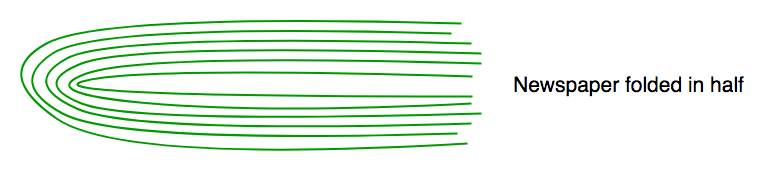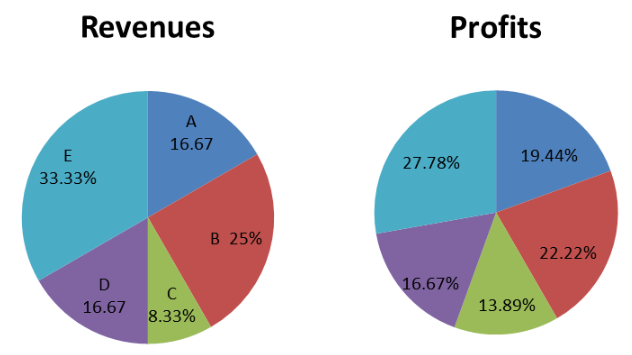# Sudo GATE 2020 Mock III (24 January 2019)

• Last Updated : 10 Nov, 2021

 Question 1

There are 6 identical toys be placed in 3 identical boxes such that no box is empty. Total number of possible ways is _______ .

 A 3 B 1 C 28 D None of these.
Sudo GATE 2020 Mock III (24 January 2019)
Discuss it

Question 1 Explanation:

Since the toys and boxes are identical, we just need to deal with a ways of splitting six into three natural numbers:

```1+2+3 = 6
1+1+4 = 6
2+2+2 = 6```

These are the 3 ways in which it can be done. Option (A) is correct.

 Question 2
The value of f∘g∘h(9) could be, if
```f(x) = 1 /x
g(x) = 1 /(x−2)
h(x) = √x ```
 A 3 B 1/3 C -5 D None of these.
Sudo GATE 2020 Mock III (24 January 2019)
Discuss it

Question 2 Explanation:
`fogoh(9) means f(g(h(9))) `
Start by solving h(9) = √9 = 3 and not (3, -3) as square root of a number is always positive. Taking the value to be 3,
```g(3) = 1(3−2) = 1
=> f(1) = 1 ```
So, option (D) is correct.
 Question 3
The Union Budget is likely to be presented on February 01, two days ahead of the ___________ date.
 A critical B conventional C suitable D convenient
Sudo GATE 2020 Mock III (24 January 2019)
Discuss it

Question 3 Explanation:
critical - expressing adverse or disapproving comments or judgements. conventional - based on or in accordance with what is generally done or believed. suitable - right or appropriate for a particular person, purpose, or situation. convenient - fitting in well with a person's needs, activities, and plans. "two days ahead of the conventional date." is correct use as the fixed date February 01 is given. Option (B) is correct.
 Question 4
Consider a three digit number abc. Then the probability such that a > b > c is __________ .
 A 720 / 900 B 180 / 900 C 120 / 900 D 150 / 900
Sudo GATE 2020 Mock III (24 January 2019)
Discuss it

Question 4 Explanation:
There are 9∗10∗10=900 three digit numbers. In the number abc, the digits are strictly decreasing. So they aren't equal. From the available ten digits, select any three. This can be done in 10C3 ways. Now arrange them in descending order. That's it. We have a favorable number. So the probability is,
`10C3 / 900 = 120 / 900 = 2 / 15 `
Option (C) is correct.
 Question 5
Chose the option with words that are not synonyms.
 A advocate, support B ability, skill C busy, active D aversion, desire
Sudo GATE 2020 Mock III (24 January 2019)
Discuss it

Question 5 Explanation:
aversion a strong dislike or disinclination. desire strongly wish for or want (e.g., liking something). Option (D) is correct.
 Question 6
If (|x| - 3) (|y| + 4) = 12. Then number of pairs of integers (x, y) satisfy this equation ?
 A 4 B 10 C 6 D 8
Sudo GATE 2020 Mock III (24 January 2019)
Discuss it

Question 6 Explanation:
Product of two integers is 12. Further |y| + 4 ±4. So, we know that one of the numbers has to be greater than 4. So, we can have,
```1 x 12 => x = ±4, y = +8
2 x 6 => x = ±5, y = +2
3 x 4 => x = ±6, y = 0
4 + 4 + 2 = 10 ```
So, there 10 possible solutions. Option (B) is correct.
 Question 7
A newspaper made of 16 large sheets of paper folded in half. The newspaper has 64 pages altogether. The first sheet contains pages 1, 2, 63, 64.If we pick up a sheet containing page number 45. What is/are the other page(s) that this sheet contains ?
 A 18 B 21 C 46 D None of these.
Sudo GATE 2020 Mock III (24 January 2019)
Discuss it

Question 7 Explanation:
Option (C) is correct. Please refer - Puzzle 28 | (Newspaper Puzzle)
 Question 8
A 4-digit number of the form aabb is a perfect square. What is the value of a - b ?
 A 3 B 2 C 1 D 4
Sudo GATE 2020 Mock III (24 January 2019)
Discuss it

Question 8 Explanation:
A number of the form aabb has to be a multiple of 11. So, it is the square of either 11 or 22 or 33 or...so on up to 99.
`882 = 7744 `
This is the only solution possible. Most of these trial and error questions need to be narrowed down a little bit before we can look for the solution. That narrowing down is critical. In this case, we should look for multiples of 11. Hence the answer is "3". Option (A) is correct.
 Question 9
India’s baffling array of state and national labor laws date to the 1940s: one provides for the type and number of spittoons in a factory. Another says an enterprise with more than 100 workers needs government permission to scale back or close. Many Indian businesses stay small in order to remain beyond the reach of the laws. Big firms use temporary workers to avoid them. Less than 15% of Indian workers have legal job security. The new government can sidestep the difficult politics of curbing privileges by establishing a new, simpler labor contract that gives basic protection to workers but makes lay-offs less costly to firms. It would apply only to new hires; the small proportion of existing workers with gold-star protections would keep them. Which of the following options best summarizes the main idea of the paragraph ?
 A More Indian workers can get permanent jobs and legal job security if existing labor laws are reformed. B Effective labor law reform can encourage many Indian businesses to grow to more than 100 workers. C Outdated Indian labor laws need to be simplified to provide basic protection to workers and curb privileges. D The difficult politics of curbing privileges can be avoided if the changes in the labor law only apply to the new hires.
Sudo GATE 2020 Mock III (24 January 2019)
Discuss it

Question 9 Explanation:
This paragraph is about dated Indian labor laws. It cites some examples highlighting how some aspects of the laws are irrelevant today, how these are affecting both firms and people they employ and suggests a possible solution to the “difficult politics of curbing privileges”. In summary, the labor laws in India are dated and need to be reformed. A simpler labor contract that gives basic protection to workers while restricting the privileges for new hires and making layoffs less costly to firms is needed. Option (C) is correct.
 Question 10
Total revenues are Rs. 1800 crores. Overall profit margin is 10%. The division with the largest revenue has the least profit margin but not the least profits. The division with the profit margin higher than all others generates the least profit. Exactly one division has the same profit margin as the overall Company. Company D generates more profits than Company E.How much profit did Company A make ?
 A Rs. 50 crores B Rs. 25 crores C Rs. 30 crores D Rs. 60 crores
Sudo GATE 2020 Mock III (24 January 2019)
Discuss it

Question 10 Explanation:
The statement “Exactly one division has the same profit margin as the overall Company.” alone is sufficient to answer it. Option (C) Rs. 30 crores is correct answer.
There are 65 questions to complete.
My Personal Notes arrow_drop_up